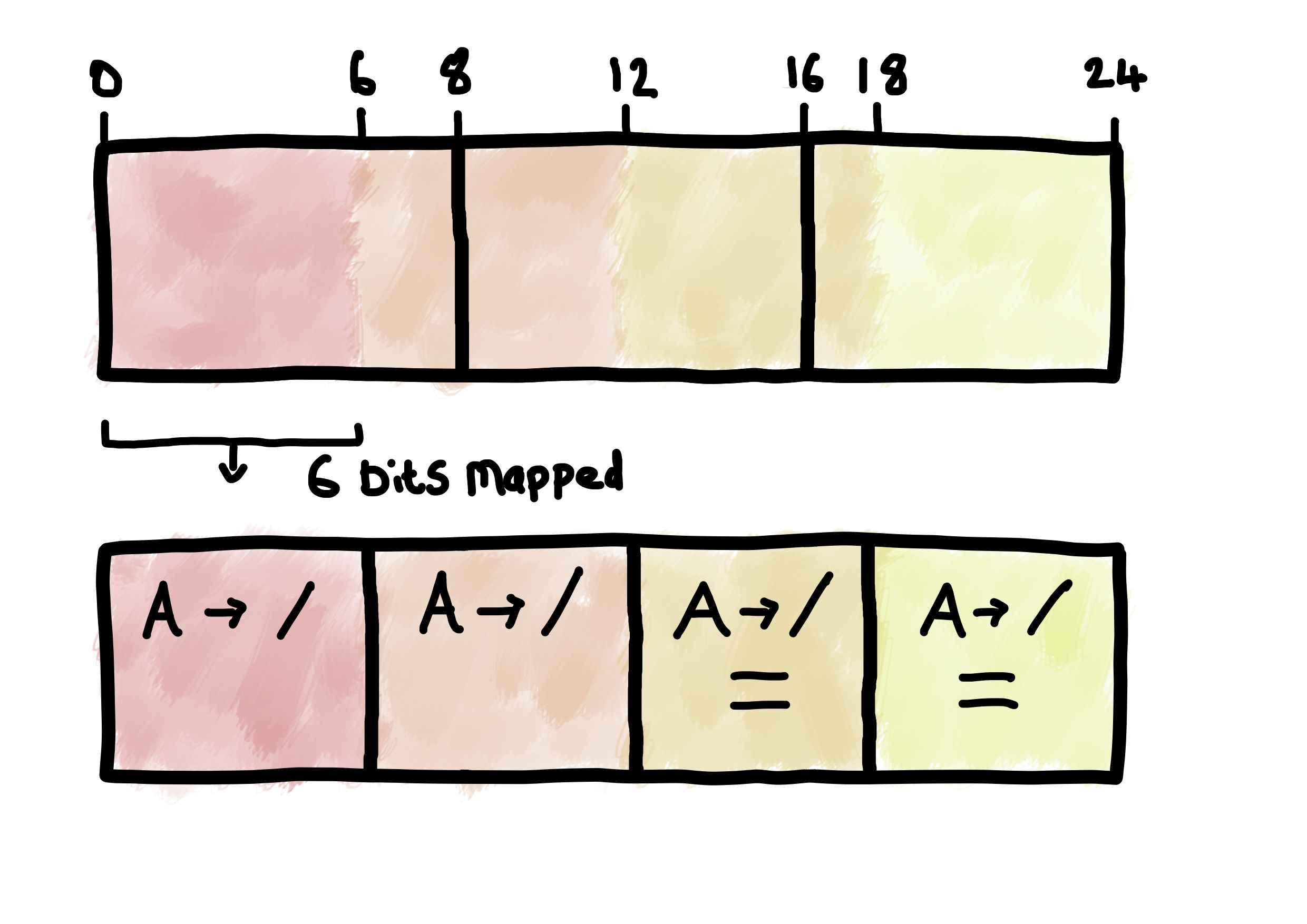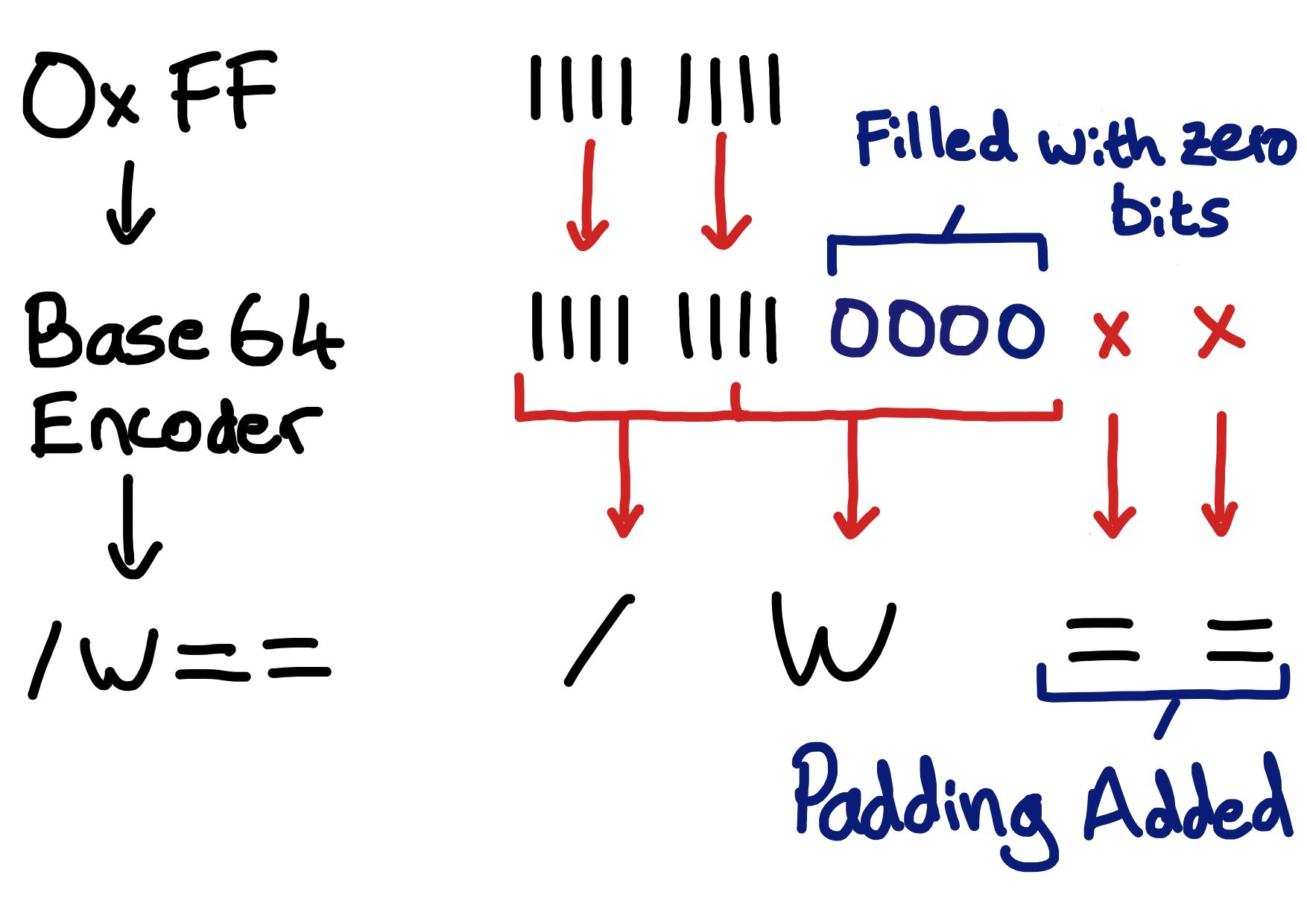# The Unterminated String

## Embedded Things and Software StuffI’ve encountered base64 encoding many times, be it in TLS certificates, SSH keys, or JWT tokens. Base64 is a useful way for holding binary data in a friendly format (“ASCII Armor”) that can be inserted into XML, JSON, or an email. Until recently, I hadn’t given much consideration to the specifics of the base64 encoding process, but after having cause to skim RFC4648 I decided to make some notes.

The base64 encoding process involves reading in 3 octets of input data, interpreting it as 4 6-bit chunks, and mapping each chunk into a base64 character.

As 6 bits can represent 64 unique values, the encoded character set has 64 characters. These are:

• `A-Z`
• `a-z`
• `0-9`
• `+`
• `/`

There is an extra “65th” character which is used to represent padding: “`=`” (see below).

Considering the decoding of data, lets say we were given the 4 character, base64 encoded string “Base”. To convert that into the original bit pattern we need to combine the binary values of each base64 character:

Base64 Character Value Binary
`B` `1` `000001`
`a` `26` `011010`
`s` `44` `101100`
`e` `30` `011110`

These four values considered sequentially are:

`000001 011010 101100 011110`

or when considered as three octets:

`00000101 10101011 00011110`

which can be represented as the following hex:

`0x05 0xAB 0x1E`

Python offers a convenient way of quickly encoding / decoding base64. The following snippet encodes and subsequently decodes the data in the above example:

``````    import base64
import binascii

binary_input = binascii.unhexlify("05AB1E")

base64_encoded = base64.standard_b64encode(binary_input)
print(f"Encoded: {base64_encoded}")
base64_decoded = base64.standard_b64decode(base64_encoded)
print(f"Decoded: {base64_decoded}")
``````

The output of this program is:

``````    Encoded: b'Base'
Decoded: b'\x05\xab\x1e'
``````

Each base64 encoded group / “quantum” must contain 4 base64 characters (which represents 24 bits of data). If there isn’t 24 bits of input data available, the encoded output group will contain 1 or 2 padding characters ("`=`").

If the input was:

• 1 octet there will be 2 padding characters present
• 2 octets there will be 1 padding character present

The encoding process must zero-fill any extra bits introduced to ensure the input is an integral number of 6 bits.

The table below shows some examples of base64 encoding 1-3 octets of data:

Input Output
`00` `AA==`
`00 00` `AAA=`
`00 00 00` `AAAA`
`FF` `/w==`
`FF FF` `//8=`
`FF FF FF` `////`

The sketch below illustrates encoding `0xFF`. The encoder will need to add 4 bits of value `0` in order to provide 12 bits of input (12 being the next integer number of 6 bits from 8). At which point, 2 base64 characters can be produced from the 12 bits of available input. To bring the total number of base64 characters to 4 there then needs to be 2 padding characters added.# URL Safe Base64

There is a second version of base64 referred to as “base64url”. This variant is considered “URL and filename safe”, and was the reason I was reading the RFC in the first place.

Base64url makes a small change to the base64 alphabet providing 2 alternative characters. These are shown in the table below.

Value base64 encoding base64url encoding
62 `+` `-`
63 `/` `_`

As this is just a mapping change, you will find that some implementations simply perform a find and replace on the base64url representation to swapout the base64url characters for base64 ones. This string can then be provided to the “standard” base64 logic. For example, you can see Python’s base64url encoding/decoding process relies on its “normal” base64 logic:

As alluded to in the comment in the Python code, this approach actually means that the `urlsafe_b64decode()` function is capable of decoding the superset of both base64 and base64url.

Running the following:

``````    print(f"Decoded base64 input   : {base64.urlsafe_b64decode(b'++//')}")
print(f"Decoded base64url input: {base64.urlsafe_b64decode(b'--__')}")
``````

produces:

``````    Decoded base64 input   : b'\xfb\xef\xff'
Decoded base64url input: b'\xfb\xef\xff'
``````